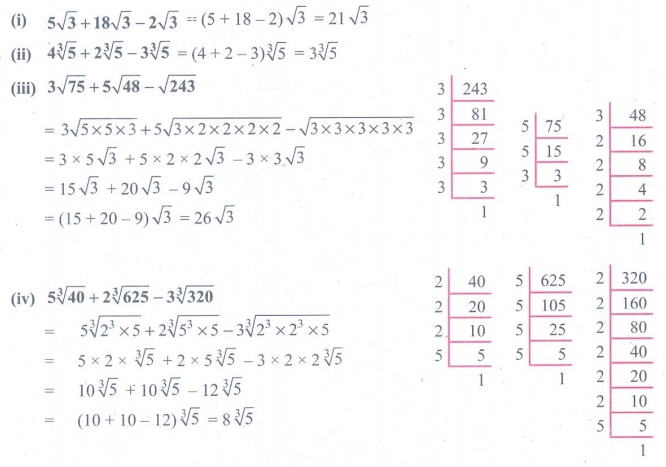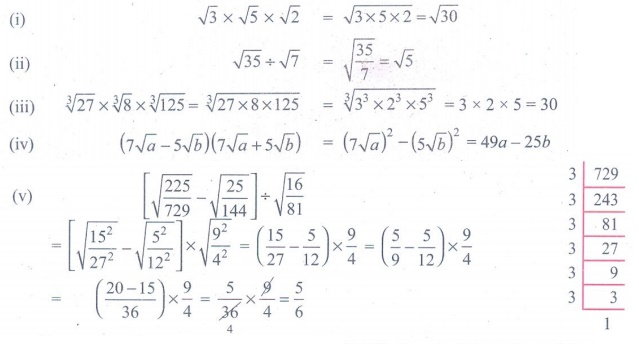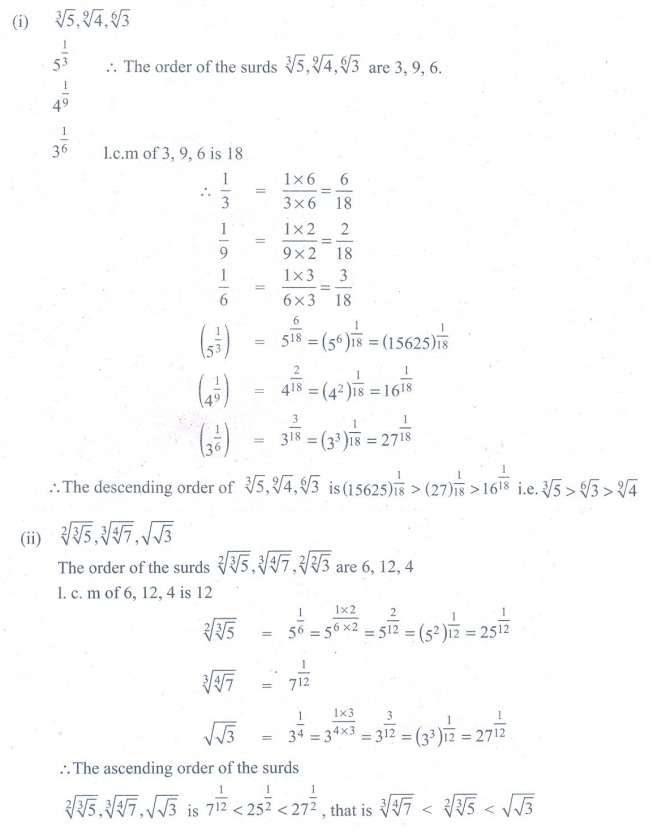Home | | Maths 9th std | Exercise 2.6 : Surds

# Exercise 2.6 : Surds

Maths : Real Numbers : Book Back, Exercise, Example Numerical Question with Answers, Solution : Exercise 2.6 : Surds

Exercise 2.6

1. Simplify the following using addition and subtraction properties of surds:

(i) 5√3 +18√3 −2√3 (ii) 4 3√5 + 2 3√5 − 3 3√5

(iii) 3√75 +5√48 − √243 (iv) 5 3√40 + 2 3√625 − 3 3√3202. Simplify the following using multiplication and division properties of surds:

(i) √3 × √5 × √2 (ii) √35 ÷ √7 (iii) 3√27 × 3√8 × 3√125 (iv) (7 a -5√b)(7√a+5√b) (v) [√(225/729) − √(25/144) ÷ √(16/81)3. If √2 = 1. 414, √3 = 1. 732, √5 = 2. 236, √10 = 3.162 , then find the values of the following correct to 3 places of decimals.

(i) √40- √20 (ii) √300 + √90 − √84. Arrange surds in descending order : (i) 3√5 , 9√4, 6√3 (ii) 23√5 , 34√7 , √√35. Can you get a pure surd when you find

(i) the sum of two surds (ii) the difference of two surds

(iii) the product of two surds (iv) the quotient of two surds

Justify each answer with an example.6. Can you get a rational number when you compute

(i) the sum of two surds (ii) the difference of two surds

(iii) the product of two surds (iv) the quotient of two surds

Justify each answer with an example.Tags : Numerical Problems with Answers, Solution | Real Numbers | Maths , 9th Maths : UNIT 2 : Real Numbers
Study Material, Lecturing Notes, Assignment, Reference, Wiki description explanation, brief detail
9th Maths : UNIT 2 : Real Numbers : Exercise 2.6 : Surds | Numerical Problems with Answers, Solution | Real Numbers | Maths